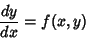## Isocline

A graphical method of solving an Ordinary Differential Equation of the formby plotting a series of curves, then drawing a curve Perpendicular to each curve such that it satisfies the initial condition. This curve is the solution to the Ordinary Differential Equation.

References

Kármán, T. von and Biot, M. A. Mathematical Methods in Engineering: An Introduction to the Mathematical Treatment of Engineering Problems. New York: McGraw-Hill, pp. 3 and 7, 1940.